## Probability Histograms

A probability histogram is a graph that shows the probability of each outcome on the $\text{y}$-axis.

### Learning Objectives

Explain the significance of a histogram as a graphical representation of data distribution

### Key Takeaways

#### Key Points

• In a probability histogram, the height of each bar showsthe true probability of each outcome if there were to be a very large number of trials (not the actual relative frequencies determined by actually conducting an experiment ).
• By looking at a probability histogram, one can visually see if it follows a certain distribution, such as the normal distribution.
• As in all probability distributions, the probabilities of all the outcomes must add up to one.

#### Key Terms

• independent: not dependent; not contingent or depending on something else; free
• discrete random variable: obtained by counting values for which there are no in-between values, such as the integers 0, 1, 2, ….

### Histograms

When examining data, it is often best to create a graphical representation of the distribution. Visual graphs, such as histograms, help one to easily see a few very important characteristics about the data, such as its overall pattern, striking deviations from that pattern, and its shape, center, and spread.

A histogram is particularly useful when there is a large number of observations. Histograms break the range of values in classes, and display only the count or percent of the observations that fall into each class. Regular histograms have a $\text{y}$-axis that is labeled with frequency. Relative frequency histograms instead have relative frequencies on the $\text{y}$-axis, with data taken from a real experiment. This chapter will focus specifically on probability histograms, which is an idealization of the relative frequency distribution.

### Probability Histograms

Probability histograms are similar to relative frequency histograms in that the $\text{y}$-axis is labeled with probabilities, but there are some difference to be noted. In a probability histogram, the height of each bar shows the true probability of each outcome if there were to be a very large number of trials (not the actual relative frequencies determined by actually conducting an experiment). Because the heights are all probabilities, they must add up to one. Think of these probability histograms as idealized pictures of the results of an experiment. Simply looking at probability histograms make it easy to see what kind of distribution the data follow.

Let’s look at the following example. Suppose we want to create a probability histogram for the discrete random variable $\text{X}$ that represents the number of heads in four tosses of a coin. Let’s say the coin is balanced, and each toss is independent of all the other tosses.

We know the random variable $\text{X}$ can take on the values of 0, 1, 2, 3, or 4. For $\text{X}$ to take on the value of 0, no heads would show up, meaning four tails would show up. Let’s call this TTTT. For $\text{X}$ to take on the value of 1, we could have four different scenarios: HTTT, THTT, TTHT, or TTTH. For $\text{X}$ to take on a value of 2, we have six scenarios: HHTT, HTHT, HTTH, THHT, THTH, or TTHH. For $\text{X}$ to take on 3, we have: HHHT, HHTH, HTHH, or THHH. And finally, for $\text{X}$ to take on 4, we only have one scenario: HHHH.

There are sixteen different possibilities when tossing a coin four times. The probability of each outcome is equal to $\frac{1}{16}=0.0625$. The probability of each of the random variables $\text{X}$ is as follows:

$\displaystyle {\text{P}(\text{X}=0) = \frac{1}{16} = 0.0625 \\ \text{P}(\text{X}=1) = \frac{4}{16} = 0.25 \\ \text{P}(\text{X}=2) = \frac{6}{16} = 0.375 \\ \text{P}(\text{X}=3) = \frac{4}{16} = 0.25 \\ \text{P}(\text{X}=4) = \frac{1}{16} = 0.0625 \\}$

Notice that just like in any other probability distribution, the probabilities all add up to one.

To then create a probability histogram for this distribution, we would first draw two axes. The $\text{y}$-axis would be labeled with probabilities in decimal form. The $\text{X}$-axis would be labeled with the possible values of the random variable $\text{X}$: in this case, 0, 1, 2, 3, and 4. Then, rectangles of equal widths should be drawn according to their corresponding probabilities.

Notice that this particular probability histogram is symmetric, and resembles the normal distribution. If we had instead tossed a coin four times in many trials and created a relative frequency histogram, we would have gotten a graph that looks similar to this one, but it would be unlikely that it would be perfectly symmetric.

## Probability Histograms and the Normal Curve

Many different types of distributions can be approximated by the normal curve.

### Learning Objectives

Assess normality using graphical tools to interpret data

### Key Takeaways

#### Key Points

• The occurrence of the normal distribution in practical problems can be loosely classified into three categories: exactly normal distributions, approximately normal distributions, and distributions modeled as normal.
• Just by looking at a probability histogram, you can tell if it is normal by looking at its shape. If the graph is approximately bell-shaped and symmetric about the mean, you can usually assume normality.
• A normal probability plot is another method used to assess normality. The data are plotted against a theoretical normal distribution in such a way that, if the data is normal, the points should form an approximate straight line.

#### Key Terms

• normal probability plot: a graphical technique used to assess whether or not a data set is approximately normally distributed
• central limit theorem: The theorem that states: If the sum of independent identically distributed random variables has a finite variance, then it will be (approximately) normally distributed.

When constructing probability histograms, one often notices that the distribution may closely align with the normal distribution. The occurrence of the normal distribution in practical problems can be loosely classified into three categories: exactly normal distributions, approximately normal distributions, and distributions modeled as normal.

### Exactly Normal Distributions

Certain quantities in physics are distributed normally, such as:

• Velocities of the molecules in the ideal gas. More generally, velocities of the particles in any system in thermodynamic equilibrium will have normal distribution, due to the maximum entropy principle.
• Probability density function of a ground state in a quantum harmonic oscillator.
• The position of a particle that experiences diffusion.

### Approximately Normal Distributions

Approximately normal distributions occur in many situations, as explained by the central limit theorem. When the outcome is produced by a large number of small effects acting additively and independently, its distribution will be close to normal. The normal approximation will not be valid if the effects act multiplicatively (instead of additively), or if there is a single external influence that has a considerably larger magnitude than the rest of the effects.

The normal approximation can be used in counting problems, where the central limit theorem includes a discrete-to-continuum approximation and where infinitely divisible and decomposable distributions are involved. This includes Binomial random variables, which are associated with binary response variables, and Poisson random variables, which are associated with rare events.

### Assumed Normality

There are many examples of problems in real life that are assumed to be normal. If you were to construct a probability histogram of these events with many trials, the histogram would appear to be bell-shaped. Examples include:

• Certain physiological measurements, such as blood pressure of adult humans.
• Measurement errors in physical experiments. This use of a normal distribution does not imply that one is assuming the measurement errors are normally distributed, rather using the normal distribution produces the most conservative predictions possible given only knowledge about the mean and variance of the errors.
• Results of standardized testing.

### How to Assess Normality

How can we tell if data in a probability histogram are normal, or at least approximately normal? The most obvious way is to look at the histogram itself. If the graph is approximately bell-shaped and symmetric about the mean, you can usually assume normality.

There is another method, however, than can help: a normal probability plot. A normal probability plot is a graphical technique for normality testing–assessing whether or not a data set is approximately normally distributed. The data are plotted against a theoretical normal distribution in such a way that the points should form an approximate straight line (, ). Departures from this straight line indicate departures from normality (, ). It is important to remember not to overreact to minor wiggles in the plot. These plots are not often produced by hand, but rather by technological tools such as the graphing calculator.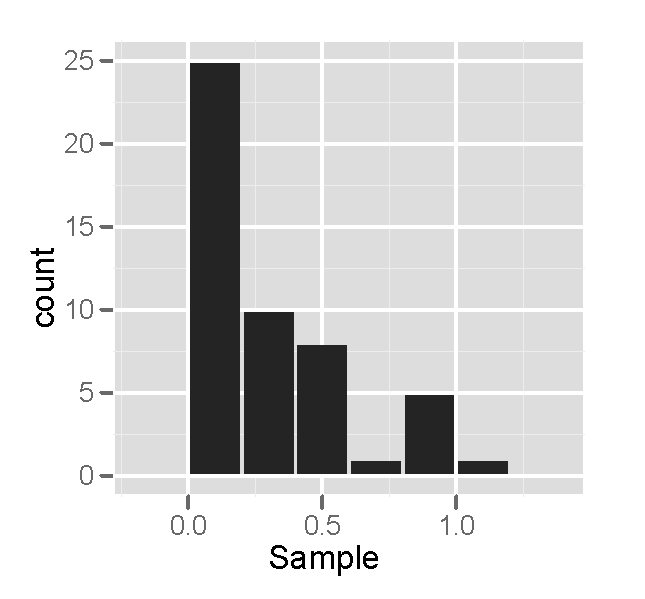Non-Normality – Histogram: This is a sample of size 50 from a right-skewed distribution, plotted as a histogram. Notice that the histogram is not bell-shaped, indicating that the distribution is not normal.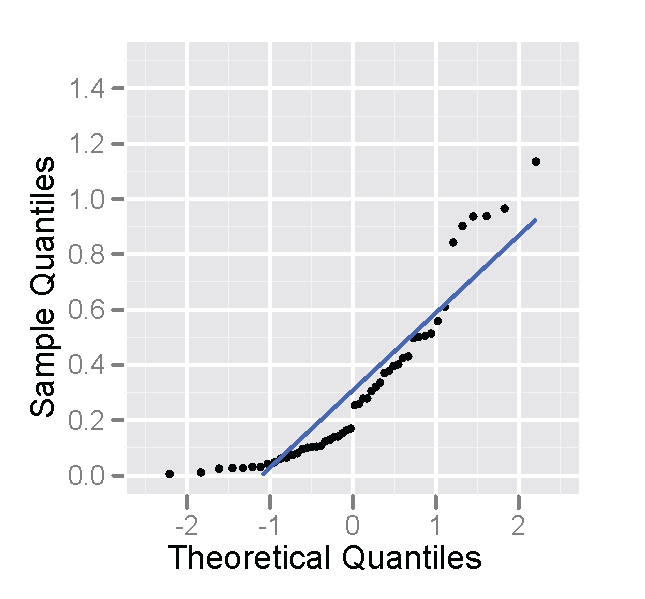Non-Normality – Probability Plot: This is a sample of size 50 from a right-skewed distribution, plotted as a normal probability plot. Notice that the points deviate on the, indicating the distribution is not normal.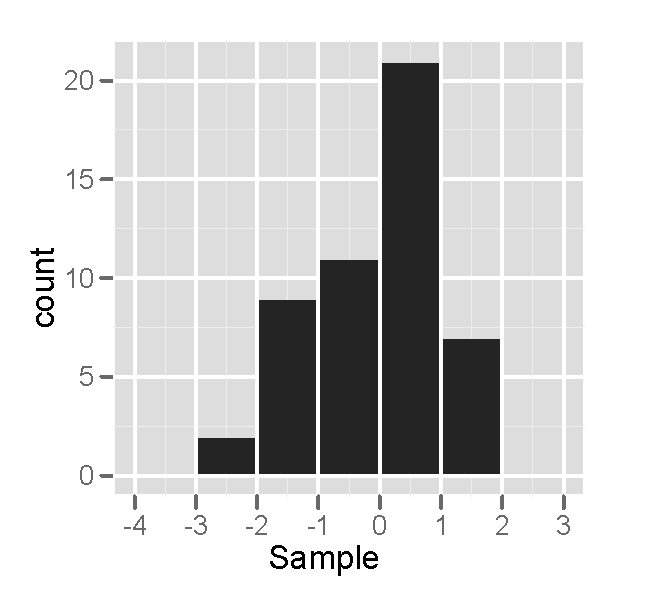Approximately Normal – Histogram: This is a sample of size 50 from a normal distribution, plotted out as a histogram. The histogram looks somewhat bell-shaped, indicating normality.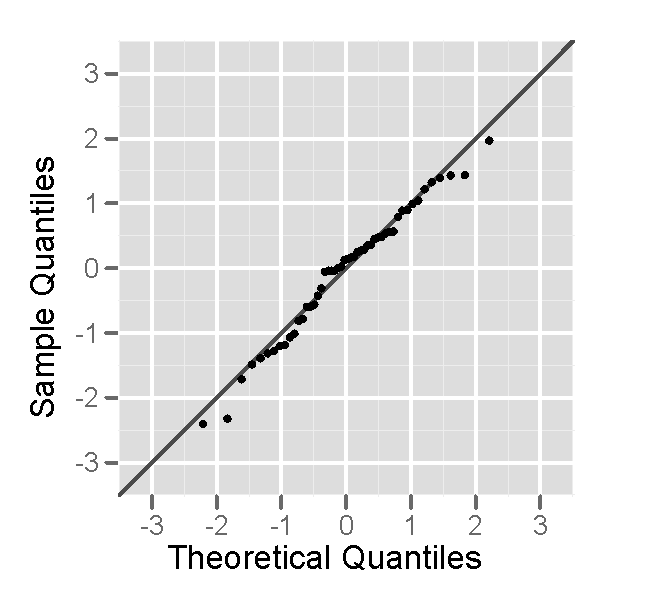Approximately Normal – Probability Plot: This is a sample of size 50 from a normal distribution, plotted as a normal probability plot. The plot looks fairly straight, indicating normality.

## Conclusion

Many distributions in real life can be approximated using normal distribution.

### Learning Objectives

Explain how a probability histogram is used to normality of data

### Key Takeaways

#### Key Points

• In a probability histogram, the height of each bar shows the true probability of each outcome if there were a very large number of trials (not the actual relative frequencies determined by actually conducting an experiment ).
• The most obvious way to tell if a distribution is approximately normal is to look at the histogram itself. If the graph is approximately bell-shaped and symmetric about the mean, you can usually assume normality.
• The normal probability plot is a graphical technique for normality testing. The data are plotted against a theoretical normal distribution in such a way that the points form an approximate straight line.
• Many things in real life are approximately normally distributed, including people’s heights and blood pressure.

#### Key Terms

• normal probability plot: a graphical technique used to assess whether or not a data set is approximately normally distributed

### What is a Probability Histogram?

It is often useful to display the data collected in an experiment in the form of a histogram. Having a graphical representation is helpful because it allows the researcher to visualize what shape the distribution takes.

Probability histograms are similar to relative frequency histograms in that the Y-axis is labeled with probabilities, but there are some differences to be noted. In a probability histogram, the height of each bar shows the true probability of each outcome if there were to be a very large number of trials (not the actual relative frequencies determined by actually conducting an experiment). Because the heights are all probabilities, they must add up to one. Think of these probability histograms as idealized pictures of the results of an experiment. Simply looking at probability histograms makes it easy to see what kind of distribution the data follow.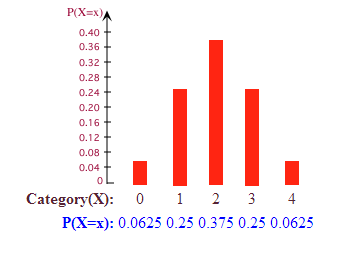Probability Histogram: This probability histogram shows the probabilities that 0, 1, 2, 3, or 4 heads will show up on four tosses of a fair coin.

### How Can We Tell If the Data is Approximately Normal?

The above example of a probability histogram is an example of one that is normal. How can we tell? The most obvious way is to look at the histogram itself. If the graph is approximately bell-shaped and symmetric about the mean, you can usually assume normality.

There is another method, however, than can help: a normal probability plot. A normal probability plot is a graphical technique for normality testing–assessing whether or not a data set is approximately normally distributed. The data are plotted against a theoretical normal distribution in such a way that the points form an approximate straight line. Departures from this straight line indicate departures from normality. It is important to remember not to overreact to minor wiggles in the plot. These plots are not often produced by hand, but rather by technological tools such as a graphing calculator.Normal Probability Plot: The data points do not deviate far from the straight line, so we can assume the distribution is approximately normal.

### Approximately Normal Distributions in Real Life

We study the normal distribution extensively because many things in real life closely approximate the normal distribution, including:

• The heights of people
• The sizes of things produced by machines
• Errors in measurements
• Blood pressure
• Scores on a standardized test